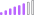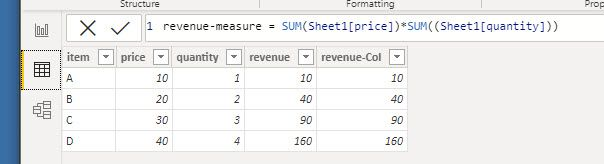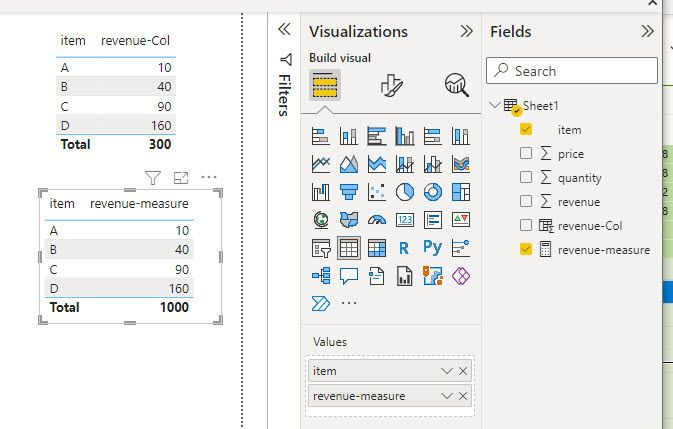cancel
Showing results for
Did you mean:

Fabric is Generally Available. Browse Fabric Presentations. Work towards your Fabric certification with the Cloud Skills Challenge.Post Patron

## measure vs column

Hi, I created measure and then a column but to my surprise they are the same when I created reports. They both sliced per item. So I do not see any difference except the Total. Why is that?

Thank you very much.

revenue-measure = SUM(Sheet1[price])*SUM((Sheet1[quantity]))

revenue-Col = Sheet1[price]*Sheet1[quantity]1 ACCEPTED SOLUTIONSuper User

This is expected, there is nothing to aggregate when you are at the lowest granularity (or say row-level )

hence, sheet(price)=sum(sheet(price))   -----

As mentioned by @bcharger 1000 is nothing but (10+20+30+40) * (1+2+3+4)=100*10=1000 because the measure will aggregate since it has got a chance to summarise for the 4 rows while for row-level it will sum up the values you see at the rows

See it this way,

----EXPLANATION OF THE DIFF IN THE TOTAL PART -------

TOTAL (SUM(PRICE) * SUM(QUANTITY))  ------

>>> SUM(PRICE)Let me first aggregate for the 4 rows

>>>SUM(QUANTITY)  Let me first aggregate for the 4 rows

>>> Now do the multiplication

>>> Now TOTAL of the same

While in the former case

its PRICE * QUANTITY (No Sum) hence you get the individual rows multiplication

At the end you do the TOTAL

That set

Regards,

Ritesh

Regards,

Ritesh

4 REPLIES 4Super User

Please mark the relevant answer as correct and help the community as well

RiteshCommunity Support

Hi @lastnn30 ,

This brings us to the difference between measure and column.

Even if they look similar, there is a big difference between calculated columns and measures. The value of a calculated column is computed during data refresh and uses the current row as a context; it does not depend on user interaction in the report. A measure operates on aggregations of data defined by the current context, which depends on the filter applied in the report – such as slicer, rows, and columns selection in a pivot table, or axes and filters applied to a chart.

At this point, you might be wondering when to use calculated columns over measures. Sometimes either is an option, but in most situations your computation needs determine your choice.

You have to define a calculated column whenever you want to do the following:

• Place the calculated results in a slicer, or see results in rows or columns in a pivot table (as opposed to the values area), or in the axes of a chart, or use the result as a filter condition in a DAX query.
• Define an expression that is strictly bound to the current row. For example, Price * Quantity cannot work on an average or on a sum of the two columns.
• Categorize text or numbers. For example, a range of values for a measure, a range of ages of customers, such as 0–18, 18–25, and so on.

However, you must define a measure whenever you want to display resulting calculation values that reflect user selections and see them in the values area of a pivot table, or in the plot area of a chart – for example:

• When you calculate profit percentage on a certain selection of data.
• When you calculate ratios of a product compared to all products but keeping the filter both by year and region.

Here is a simple sample, please refer to it to see if it helps you.

Best Regards

Community Support Team _ Polly

If this post helps, then please consider Accept it as the solution to help the other members find it more quickly.Super User

This is expected, there is nothing to aggregate when you are at the lowest granularity (or say row-level )

hence, sheet(price)=sum(sheet(price))   -----

As mentioned by @bcharger 1000 is nothing but (10+20+30+40) * (1+2+3+4)=100*10=1000 because the measure will aggregate since it has got a chance to summarise for the 4 rows while for row-level it will sum up the values you see at the rows

See it this way,

----EXPLANATION OF THE DIFF IN THE TOTAL PART -------

TOTAL (SUM(PRICE) * SUM(QUANTITY))  ------

>>> SUM(PRICE)Let me first aggregate for the 4 rows

>>>SUM(QUANTITY)  Let me first aggregate for the 4 rows

>>> Now do the multiplication

>>> Now TOTAL of the same

While in the former case

its PRICE * QUANTITY (No Sum) hence you get the individual rows multiplication

At the end you do the TOTAL

That set

Regards,

Ritesh

Regards,

RiteshPost Prodigy

I believe; The measure is summing the price column (100) and summing the quantity column (10) then multiplying them to arrive at 1,000. The calculated column is looking at each row but not the aggregate.Announcements#### Power BI Monthly Update - November 2023

Check out the November 2023 Power BI update to learn about new features.#### Fabric Community News unified experience

Read the latest Fabric Community announcements, including updates on Power BI, Synapse, Data Factory and Data Activator.#### The largest Power BI and Fabric virtual conference

130+ sessions, 130+ speakers, Product managers, MVPs, and experts. All about Power BI and Fabric. Attend online or watch the recordings.Top Solution Authors
Top Kudoed Authors
Users online (2,111)